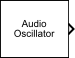# Audio Oscillator

Generate sine, square, and sawtooth waveforms

• Library:
• Audio Toolbox / Sources

•## Description

The Audio Oscillator block generates tunable waveforms. Typical uses include the generation of test signals for test benches, and the generation of control signals for audio effects. Parameters of the Audio Oscillator block specify the type of waveform generated.## Ports

### Input

expand all

#### Dependencies

To enable this port, select Specify frequency from input port for the Frequency (Hz) parameter.

Data Types: `single` | `double`

#### Dependencies

To enable this port, select Specify amplitude from input port for the Amplitude parameter.

Data Types: `single` | `double`

#### Dependencies

To enable this port, select Specify DC offset from input port for the DC offset parameter.

Data Types: `single` | `double`

### Output

expand all

The Audio Oscillator block outputs a periodic signal defined by the parameters of the block.

Data Types: `single` | `double`

## Parameters

expand all

If a parameter is listed as tunable, then you can change its value during simulation.

The waveforms are generated using the algorithms specified by the `sin`, `square`, and `sawtooth` functions.

• If Signal type is set to `sine`, specify Frequency (Hz) as a scalar or as a vector. If Frequency (Hz) is set to an N-element vector, then the output from the block is the single-channel sum of N sinusoids. If Frequency (Hz) is set to an N-element vector, then Amplitude, Phase offset, and DC offset must be scalars or N-element vectors.

• For square waveforms, specify Frequency (Hz) as a scalar.

• For sawtooth waveforms, specify Frequency (Hz) as a scalar.

To specify Frequency (Hz) from an input port, select Specify frequency from input port.

Tunable: Yes

• If Signal type is set to `sine`, specify Amplitude as a scalar or as a vector. If Amplitude is set to an N-element vector, then the output from the block is the single-channel sum of N sinusoids. If Amplitude is set to an N-element vector, then Frequency (Hz), Phase offset, and DC offset must be scalars or N-element vectors.

• For square waveforms, specify Amplitude as a scalar.

• For sawtooth waveforms, specify Amplitude as a scalar.

To specify Amplitude from an input port, select Specify amplitude from input port.

Tunable: Yes

The phase offset range, `[0,1]`, corresponds to a normalized 2π radians interval.

• If Signal type is set to `sine`, specify Phase offset as a scalar or as a vector. If Phase offset is set to an N-element vector, then the output from the block is the single-channel sum of N sinusoids. If Phase offset is set to an N-element vector, then Frequency (Hz), Amplitude, and DC offset must be scalars or N-element vectors.

• For square waveforms, specify Amplitude as a scalar.

• For sawtooth waveforms, specify Amplitude as a scalar.

• If Signal type is set to `sine`, specify DC offset as a scalar or as a vector. If DC offset is set to an N-element vector, then the output from the block is the single-channel sum of N sinusoids. If DC offset is set to an N-element vector, then Frequency (Hz), Amplitude, and Phase offset must be scalars or N-element vectors.

• For square waveforms, specify Amplitude as a scalar.

• For sawtooth waveforms, specify Amplitude as a scalar.

To specify DC offset from an input port, select Specify DC offset from input port.

Tunable: Yes

Square waveform duty cycle is the percentage of one period in which the waveform is above the median amplitude. A duty cycle value of 1 or 0 is equivalent to a DC signal.

#### Dependencies

To enable this parameter, set Signal type to `square`.

Sawtooth width determines the point in a sawtooth waveform period at which the maximum occurs.

#### Dependencies

To enable this property, set Signal type to `sawtooth`.

Number of samples per frame, specified as a positive integer.

The sample rate must be greater than twice the value specified in Frequency (Hz).

Data type of generated waveform, specified as `double` or `single`.

Tunable: No

• `Code generation` – Simulate the model using generated C code. The first time you run a simulation, Simulink® generates C code for the block. The C code is reused for subsequent simulations, as long as the model does not change. This option requires additional startup time, but the speed of the subsequent simulations is comparable to `Interpreted execution`.

• `Interpreted execution` – Simulate model using the MATLAB® interpreter. This option reduces startup time and the simulation has speed comparable to `Code generation`. In this mode, you can debug the source code of the block.

## Block Characteristics

 Data Types `double` | `single` Direct Feedthrough `no` Multidimensional Signals `no` Variable-Size Signals `no` Zero-Crossing Detection `no`

## Version History

Introduced in R2020a